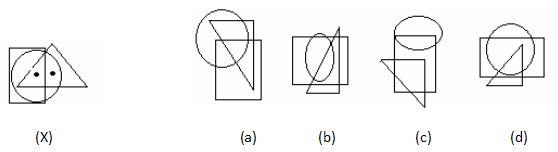# Dot Situation - Non Verbal Reasoning questions

## Dot Situation - Non Verbal Reasoning questions

1. Select the figure which satisfies the same conditions of placement of the dots as in fig. (X).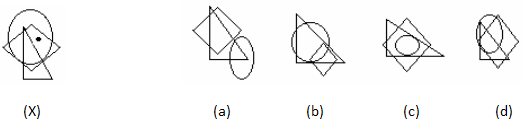2. Select the figure which satisfies the same conditions of placement of the dots as in fig. (X).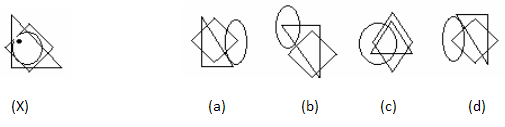3. Select the figure which satisfies the same conditions of placement of the dots as in fig. (X).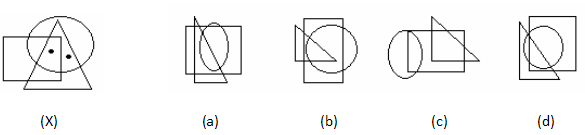4. Select the figure which satisfies the same conditions of placement of the dots as in fig. (X).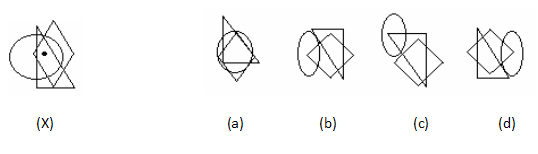5. Select the figure which satisfies the same conditions of placement of the dots as in fig. (X).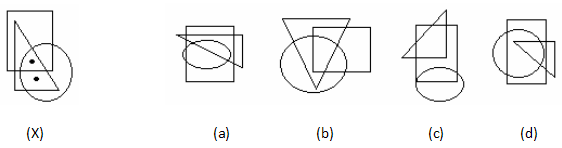6. Select the figure which satisfies the same conditions of placement of the dots as in fig. (X).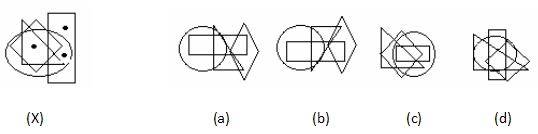7. Select the figure which satisfies the same conditions of placement of the dots as in fig. (X).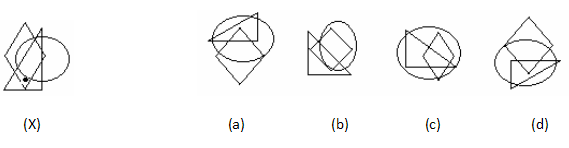8. Select the figure which satisfies the same conditions of placement of the dots as in fig. (X).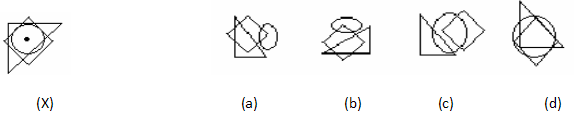9. Select the figure which satisfies the same conditions of placement of the dots as in fig. (X).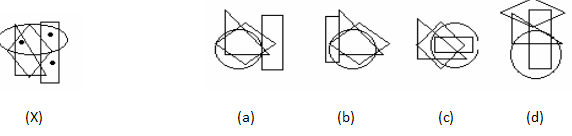10. Select the figure which satisfies the same conditions of placement of the dots as in fig. (X).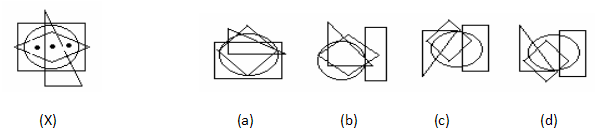11. Select the figure which satisfies the same conditions of placement of the dots as in fig. (X).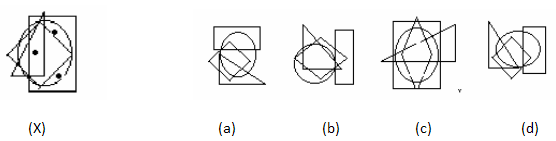12. Select the figure which satisfies the same conditions of placement of the dots as in fig. (X).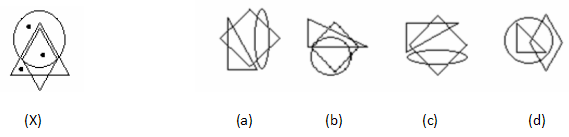13. Select the figure which satisfies the same conditions of placement of the dots as in fig. (X).14. Select the figure which satisfies the same conditions of placement of the dots as in fig. (X).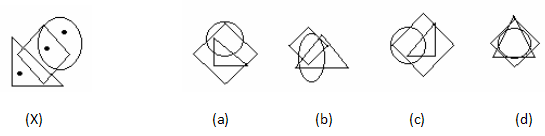15. Select the figure which satisfies the same conditions of placement of the dots as in fig. (X).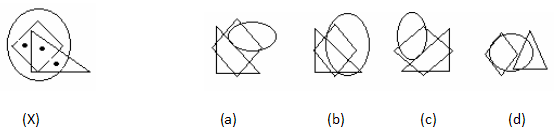16. Select the figure which satisfies the same conditions of placement of the dots as in fig. (X).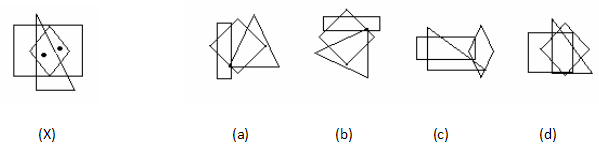17. Select the figure which satisfies the same conditions of placement of the dots as in fig. (X).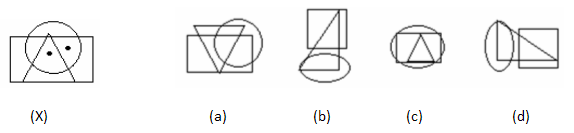18. Select the figure which satisfies the same conditions of placement of the dots as in fig. (X).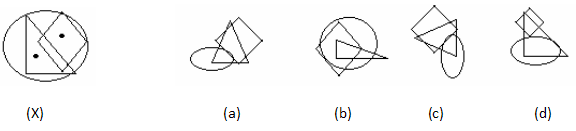19. Select the figure which satisfies the same conditions of placement of the dots as in fig. (X).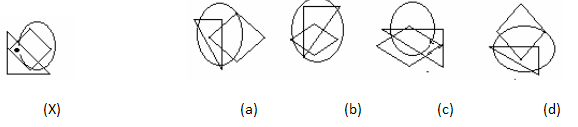20. Select the figure which satisfies the same conditions of placement of the dots as in fig. (X).